# Excel Applications for Accounting Principles

## Quiz 9 :Depreciation DeprecLooking for Accounting Homework Help?

## Quiz 9 :Depreciation DeprecPROBLEM DATA Dunedin Drilling Company recently acquired a new machine at a cost of $350,000. The machine has an estimated useful life of four years or 100,000 hours, and a salvage value of$30,000. This machine will be used 30,000 hours during Year 1, 20,000 hours in Year 2, 40,000 hours in Year 3, and 10,000 hours in Year 4. REQUIREMENT Dunedin buys equipment frequently and wants to print a depreciation schedule for each asset's life. Review the worksheet called DEPREC that follows these requirements. Since some assets acquired are depreciated by straight-line, others by units of production, and others by double-declining balance, DEPREC shows all three methods. You are to use this worksheet to prepare depreciation schedules for the new machine.
Free
Essay

Straight - Line Depreciation:
Depreciation under this method is calculated by dividing cost less salvage value by useful life (number of years). Under straight-line method of depreciation, same percentage of asset cost is allocated for the accounting year.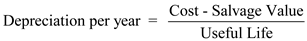Units - of - Production Method:
Activity method of depreciation is also known as units-of-production method which assumes that depreciation is calculated based on use of asset or productivity instead of passage of time. Depreciation is calculated based on actual number of hours it worked or number of units it produces. But depreciation based on the number of machine hours.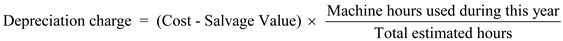Double-Declining Balance method :
A depreciation method which is dependent on a fixed percentage of value reduction of assets over its service life.
This method uses a depreciation rate which indicates some multiple of straight-line method.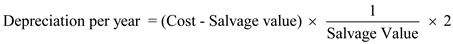Compute depreciation under different methods using the worksheet as follows: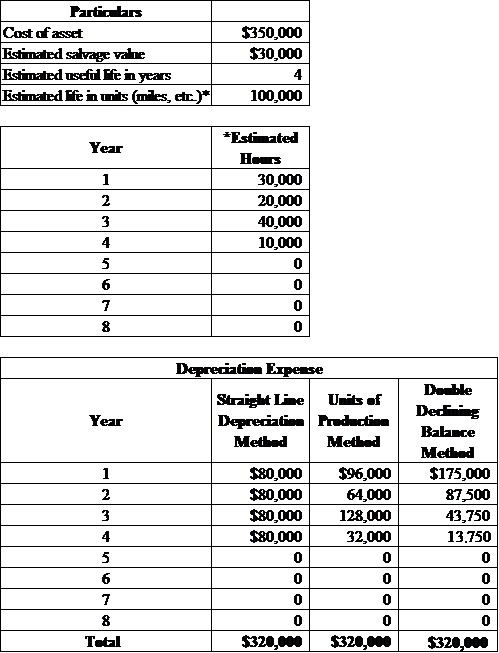Working note:
Straight-Line Method:Hence, depreciation for each year is $80,000. Units - of - Production Method: Year 1:Year 2:Year 3:Year 4:Double - Declining Balance Method: Year 1:Year 2:Year 3:Year 4: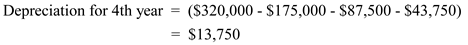PROBLEM DATA Dunedin Drilling Company recently acquired a new machine at a cost of$350,000. The machine has an estimated useful life of four years or 100,000 hours, and a salvage value of $30,000. This machine will be used 30,000 hours during Year 1, 20,000 hours in Year 2, 40,000 hours in Year 3, and 10,000 hours in Year 4. REQUIREMENT Open the file DEPREC from the website for this book at cengagebrain.com. The formulas on this worksheet are somewhat complicated. Be sure to use cell references wherever possible in your formulas instead of numbers. You should find the year numbers in column B helpful for some of the formulas in columns C, D, and E. Your instructor may tell you whether you are to construct your own formulas or use =SLN and =DDB (see Appendix A of Excel Quick for an explanation of these functions). For example, FORMULA1 could be either =(D7-D8)/D9 or =SLN(D7,D8,D9). Assume that all assets acquired will have at least a three-year life. Note that all cells on the worksheet containing zeros have been preprogrammed to perform depreciation calculations. Enter the formulas in the appropriate cells. Does your depreciation total$320,000 under all three methods If not, correct your error. ( Hint: If your double-declining-balance method is off, check cell E32 where FORMULA12 is located. It should include an =IF statement that will enter a modified calculation of depreciation if Year 4 is the last year of the asset's expected life. See Appendix A of Excel Quick for an explanation of the =IF function. Save the completed file as DEPREC2. Print the worksheet. Also print your formulas using landscape orientation and fit-to-1 page scaling. Check figure: DDB depreciation for Year 3 (cell E31), $43,750. Free Essay Answer: Answer: In the open worksheet, enter the formulas to calculate depreciation for each year under each method. Use cell references instead of numbers when available so that the spreadsheet updates automatically when any figure is changed. To calculate depreciation using the straight line method, take the cost of the asset less the salvage value and divide it by the number of years of its useful life. You will have the same figure for depreciation for each year. Using the units of production depreciation method, the depreciation is calculated based on the number of units used during that period. To calculate this, subtract the salvage value from the cost of the asset and then divide the total estimated units of production for the life of the asset. You will use that per unit cost and multiply by the estimated units used for the period to determine depreciation cost. The double declining method is used when the depreciation of the asset will be highest during its first few years of life. This value is calculated by 2x the depreciation rate of the straight line method multiplied by the book value at the beginning of the year. When you finish inputting all the formulas, your worksheet should look like this: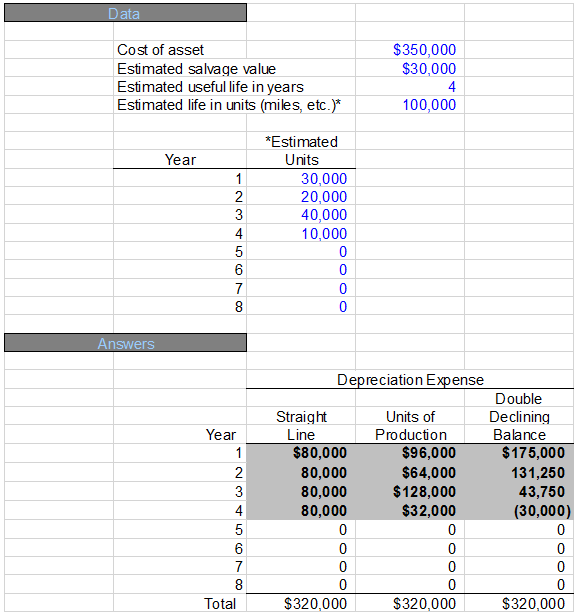PROBLEM DATA Dunedin Drilling Company recently acquired a new machine at a cost of$350,000. The machine has an estimated useful life of four years or 100,000 hours, and a salvage value of $30,000. This machine will be used 30,000 hours during Year 1, 20,000 hours in Year 2, 40,000 hours in Year 3, and 10,000 hours in Year 4. REQUIREMENT In the space below, prepare the journal entry to record the depreciation taken in Year 3 under the units of production method. Free Essay Answer: Answer: At the end of the period or fiscal year, depreciation needs to be recorded in the books for that year's depreciable assets. The journal entry would be a debit to depreciation expense and a credit to accumulated depreciation. If the depreciation for an asset was$128,000 for a year, the following journal entry should be prepared: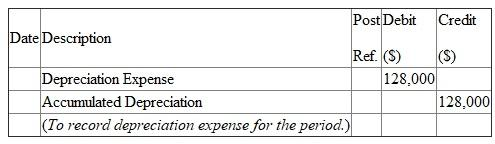WHAT-IF ANALYSIS To test your formulas, assume the machine purchased had an estimated useful life of three years (20,000, 30,000, and 50,000 hours, respectively). Enter the new information in the Data Section of the worksheet. Does your depreciation total $320,000 under all three methods There are three common errors made by students completing this worksheet. Let's clear up two of them. One, an asset that has a three-year life should have no depreciation claimed in Year 4. This can be corrected using an =IF statement in Year 4. For example, the correct formula in cell C32 is =IF(B32 D9,0,(D7-D8)/D9) or =IF(B32 D9,0,SLN(D7,D8,D9)). You may wish to edit what you have already entered rather than retype it. Two, as mentioned in requirement 2, the double-declining-balance calculation needs to be modified in the last year of the asset's life. Assuming you have already modified the formula for Year 4 (per instructions in step 2), alter the formula for Year 3 also. If you corrected any formulas, test their correctness by trying different estimated useful lives (between 3 and 8) in cell E9. Then reset the Data Section to the original values, save the revised file as DEPREC2, and reprint the worksheet to show the correct formulas. The third common error doesn't need to be corrected in this problem. The general form of the double-declining-balance formula needs to be modified to check the net book value of the asset each year to make sure it does not go below salvage value. =DDB does this automatically, but if you are writing your own formulas, this gets very complicated and is beyond the scope of the problem. Essay Answer:WHAT-IF ANALYSIS A truck was recently purchased for$75,000 with a salvage value of \$5,000 and an estimated useful life of eight years or 150,000 miles (24,000 miles per year for the first five years and 10,000 miles per year after that). Enter the new information in the Data Section of the worksheet. Again, make sure the totals for all three methods are in agreement. Print the worksheet. Save this new data as DEPREC5.
Essay